# JAC Class 10 Maths Solutions Chapter 11 Constructions Ex 11.1

Jharkhand Board JAC Class 10 Maths Solutions Chapter 11 Constructions Ex 11.1 Textbook Exercise Questions and Answers.

## JAC Board Class 10 Maths Solutions Chapter 11 Constructions Exercise 11.1

In each of the following, give the justification of the construction also:

Question 1.
Draw a line segment of length 7.6 cm and divide it in the ratio 5 : 8. Measure the two parts.
Solution :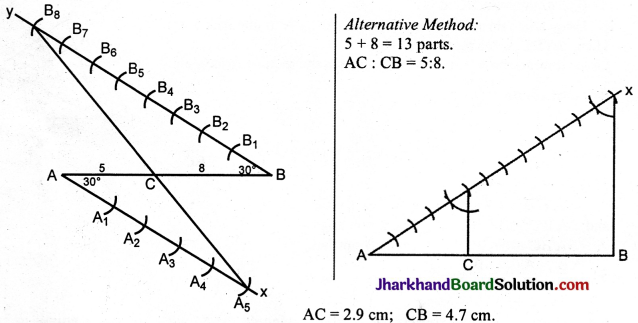Steps of construction:
1. Draw the line segment AB = 7.6 cm.
2. At A, below AB, make an angle BAx = 30°.
3. At B, above AB, make an angle ABy = 30°.
B$$\hat{A}$$x = A$$\hat{B}$$y = 30°
These are alternate angles. ∴ Ax || By.
4. With a convenient radius cut off five equal parts
AA1 = A1A2 = A2A3 = A3A4 = A4A5 in Ax.
5. With the same radius cut off eight equal parts.
BB1 = B1B2 = B2B3 = B3B4 = B4B5 = B5B6 = B6B7 = B7B8.
6. Join A5B8. Let it cut AB at C.
AC : CB = 5 : 8.

In ΔACA5 and CBB8
1. A$$\hat{C}$$A5 = B$$\hat{C}$$B8 (V.O.A.)
2. C$$\hat{A}$$A5 = A$$\hat{B}$$B8 (Alternate angles)
3. C$$\hat{A}$$5A = B$$\hat{B}$$8C (Alternate angles)
Δs are equiangular.
∴$$\frac{\mathrm{AA}_5}{\mathrm{BB}_8}=\frac{\mathrm{CA}_5}{\mathrm{AB}_8}=\frac{\mathrm{CA}}{\mathrm{BC}}$$
∴ $$\frac{\mathrm{CA}}{\mathrm{BC}}=\frac{\mathrm{AA}_5}{\mathrm{BB}_8}=\frac{5}{8}$$Question 2.
Construct a triangle of sides 4 cm, 5 cm and 6 cm and then a triangle similar to it whose sides are $$\frac{2}{3}$$ of the corresponding sides of the first triangle.
Solution :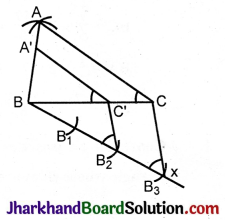Steps of construction:

1. Construct ΔABC given AB = 4 cm, BC = 5 cm, AC = cm.
2. At B, make an acute angle CBx.
3. Divide Bx into three equal parts with a convenient radius.
4. Join B3C.
5. From B2 draw a parallel to B3C.
6. Let it cut BC at C’.
7. At C’ make angle A’C’B = ACB.

Join A’C’.
∴ ΔABC ||| A’BC’.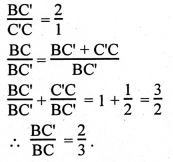In ΔABC and ΔA’BC’,
1.A$$\hat{B}$$C = A’$$\hat{B}$$C’ (Common angle)
2. A$$\hat{C}$$B = A’$$\hat{C’}$$B (Corresponding angles)
3. B$$\hat{A}$$C = B$$\hat{A’}$$C’ (Remaining angles)
Δs are equiangular.
∴ $$\frac{\mathrm{AC}}{\mathrm{A}^{\prime} \mathrm{C}^{\prime}}=\frac{\mathrm{AB}}{\mathrm{A}^{\prime} \mathrm{B}^{\prime}}=\frac{\mathrm{BC}}{\mathrm{BC} \mathrm{C}^{\prime}}$$ = $$\frac{3}{2}$$ ∴ $\frac{BC’}{BC}=\frac{2}{3}$

Question 3.
Construct a triangle with sides 5 cm, 6 cm and 7 cm and then another triangle whose sides are $$\frac{7}{5}$$ of the corresponding sides of the first triangle.
Solution :
Steps of construction:

1. Construct a triangle ABC given BC= 7 cm, AB = 5 cm, AC = 6 cm.
2. At B, below BC, make an acute angle CBx.
3. With a convenient radius cut off seven equal parts BB1 = B1B2 = B2B3 = B3B4 = B4B5 = B5B6 = B6B7.
4. Join B5C.
5. From B1 draw a parallel to B5C to cut BC produced at C’.
6. At C’ draw a parallel to CA to meet BA produced at A’.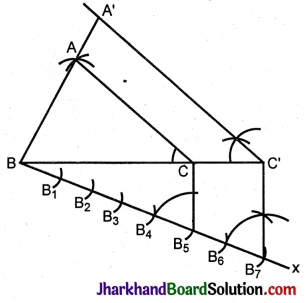A’BC’ is the required triangle.
In ΔABC and ΔA’BC’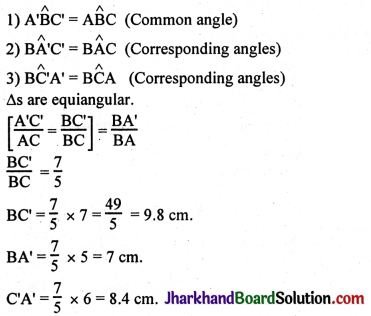Question 4.
Construct an isosceles triangle whose base is 8 cm and altitude 4 cm and then another triangle whose sides are 1$$\frac{1}{2}$$ times the corresponding sides of the isosceles triangle.
Solution :Steps of construction:

1. Draw a line segment BC = 8 cm.
2. Draw its perpendicular bisector.
3. Cut off DA = 4 cm. (altitude given)
4. Join AB and AC. ABC is the required triangle.
5. At B, below BC, draw an acute angle CBx.
6. With a convenient radius cut off three equal parts BB1 = B1B2 = B2B3.
7. Join B2C.
8. At B3 draw a parallel to B2C to meet BC extended at C’.
9. At C’ draw a parallel to CA to meet BA produced at A’.
10. Join A’C’. A’BC’ is the required triangle similar to ΔABC.

In ΔA’BC’ and ABC
1. A’$$\hat{B}$$C’ = A$$\hat{B}$$C (Common angle)
2. B$$\hat{A}$$‘C’ = B$$\hat{A}$$C (Corresponding angles)
3. A’$$\hat{C}$$‘B = A$$\hat{C}$$B
Δs are equiangular.
∴ $$\frac{\mathrm{A}^{\prime} \mathrm{C}^{\prime}}{\mathrm{AC}}=\frac{\mathrm{BC}}{\mathrm{BC}}=\frac{\mathrm{A}^{\prime} \mathrm{B}}{\mathrm{AB}}$$
$$\frac{BC’}{BC}$$ = $$\frac{3}{2}$$
BC = 8 cm, BC’ = 8 × $$\frac{3}{2}$$ = 12 cm.
BA = CA = 5.6 cm ∴ A’B = A’C’ = 5.6 × $$\frac{3}{2}$$ = 8.4 cm.

Question 5.
Draw a triangle ABC with side BC = 6 cm, AB = 5 cm and ∠ABC = 60°. Then construct a triangle whose sides are $$\frac{3}{4}$$ of the corresponding sides of the triangle ABC.
Solution :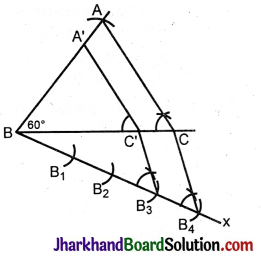Steps of construction:

1. Construct ΔABC given BC = 6 cm, AB = 5 cm, A$$\hat{B}$$C = 60°.
2. At B, below BC, make an acute angle CBx.
3. In Bx cut off four equal parts BB1 = B1B2 = B2B3 = B3B4
4. Join B4C.
5. From B3 draw a parallel to B4C to meet BC at C’.
6. At C’ draw a parallel to CA to meet CA at A’.

A’BC’ is the required triangle similar to ΔABC.
In Δs A’BC’ and ABC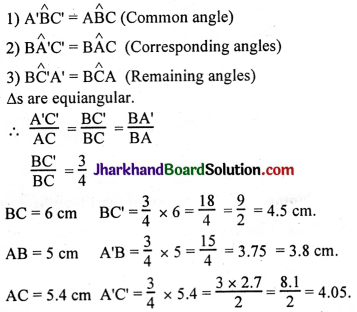Question 6.
Draw a triangle ABC with side BC = 7 cm, ∠B = 45°, ∠A = 105°. Then, construct a triangle whose sides are $$\frac{4}{3}$$ times the corresponding sides of ΔABC.
Solution :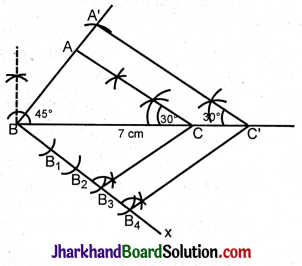$$\hat{A}$$ + $$\hat{B}$$ + $$\hat{C}$$ = 180°
$$\hat{A}$$ + $$\hat{B}$$ = 150° (105° + 45°)
∴ $$\hat{C}$$ = 30°
Steps of construction:
1. In ΔABC, BC = 7 cm.
$$\hat{B}$$ = 45°, $$\hat{C}$$ = 105°
∴ $$\hat{A}$$ = 180° – 45° – 105°
= 180° – 150° = 30°.
Construct ΔABC given BC = 7 cm, $$\hat{B}$$ = 45°, $$\hat{C}$$ = 30°.
2. At B draw an acute angle CBx.
3. In Bx cut off four equal parts BB1 = B1B2 = B2B3 = B3B4 with a convenient radius.
4. Join B3C.
5. At B4 draw a parallel to B3C to meet BC produced at C’.
6. At C’ make angle of 30° equal to A$$\hat{C}$$B. Let it meet BA produced at A’.
A’BC’ is the required triangle similar to ΔABC.
In ΔC’BB4 CB3 || C’B4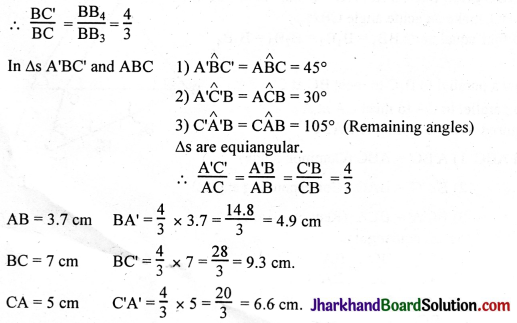Question 7.
Draw a right triangle in which the sides (other than hypotenuse) are of lengths 4 cm and 3 cm. Then construct another triangle whose sides are $$\frac{5}{3}$$ times the corresponding sides of the given triangle.
Solution :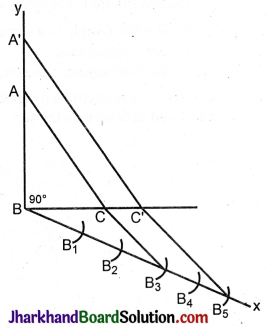BC’ = $$\frac{5}{3}$$ × BC = $$\frac{5}{3}$$ × 3 = 5 cm
BA’= $$\frac{5}{3}$$ × 4 = $$\frac{20}{3}$$ = 6.6 cm
A’C’ = $$\frac{5}{3}$$ × 5 = $$\frac{25}{3}$$ = 8.3 cm.

Steps of construction:

1. Construct ΔABC given BC= 3 cm, $$\hat{B}$$ = 90°, BA = 4 cm.
2. At B, make an acute angle CBx.
3. Cut off five equal parts BB1 = B1B2 = B2B3 = B3B4 = B4B5 along Bx with a convenient radius.
4. Join B3C.
5. At B5 draw a parallel to B3C to meet BC produced at C’.
6. At C’ draw a parallel to CA to meet BA produced at A’.

A’BC’ is the required triangle similar to ΔABC.
BC’ : BC = BB5 = BB3
$$\frac{\mathrm{BC}^{\prime}}{\mathrm{BC}}=\frac{\mathrm{BB}_5}{\mathrm{BB}_3}$$ = $$\frac{5}{3}$$

In Δs A’BC’ and ABC
1. A’$$\hat{B}$$C’ = A$$\hat{B}$$C = 90°
2. B$$\hat{A}$$‘C’ = B$$\hat{A}$$C (Corresponding angles)
3. B$$\hat{C}$$‘A’ = B$$\hat{C}$$A
Δs are equiangular.
∴ $$\frac{A’C’}{AC}$$ = $$\frac{BC’}{BC}$$ = $$\frac{BA’}{BA}$$ = $$\frac{5}{3}$$